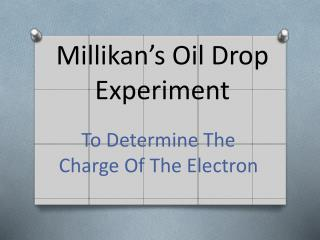DownloadDownload PresentationMillikan’s Oil Drop Experiment

# Millikan’s Oil Drop Experiment

Télécharger la présentation## Millikan’s Oil Drop Experiment

- - - - - - - - - - - - - - - - - - - - - - - - - - - E N D - - - - - - - - - - - - - - - - - - - - - - - - - - -
##### Presentation Transcript

1. Millikan’s Oil Drop Experiment To Determine The Charge Of The Electron

2. Success Criteria • Be able to state and explain Stokes Law for bodies moving through fluids • Be able to identify the forces acting on a stationary charged object in an electric field • Recall and explain how Millikan was experimentally able to determine the charge on the electron

3. Falling through a fluid • Give a detailed description of the forces acting on an object moving in a fluid • How could the drag force on the object be calculated?

4. Measuring the viscosity of water • Measurements: • Mass of ball bearing • Radius of ball bearing • Terminal velocity of ball bearing

5. Stoke’s Law • When an object is dropped through a fluid, it experiences a force called viscous drag. • This force acts in the opposite direction to the velocity of the object, and is due to the viscosity of the fluid. • The force on a spherical object can be calculated using Stoke’s Law: F=6πηrv η=coeff of viscosity of fluid, r=radius of object v=velocity of object, π=Pi

6. Assumptions • Small Reynolds number (small particles satisfy this) • Laminar flow • Smooth, spherical particles • Homogeneous fluid • Particles do not interfere with each other

7. Millikan’s Apparatus • Atomiser created a fine mist of oil droplets, charged by friction (Later experiments used x-rays). • Some droplets fell through hole and could be viewed through the microscope with a scale to measure distances and hence velocities. • Millikan applied an electric field between the plates which would exert a force on the droplets. He could adjust the p.d. and hence field strength to get the oil droplets to hover.

8. Forces without a field Before the electric field is turned on, the forces on the droplets are: a) the weight of the drop; b) the viscous force from the air. The drop will reach terminal velocity when the two forces are equal. mg=6πηrv The mass of the drop is the volume multiplied by the density of the oil, ρ , so the equation can be written: 4/3πr3ρg = 6πηrv => r2 = 9ηv/2ρg Millikan in earlier experiments measured η and ρ, and now can calculate r. Viscous Force Weight

9. With An Electric Field Millikan adjusted p.d. till the drop was stationary. Since for viscous force to act the drop needed to be moving, this for is now not there. Two forces now are: • Weight of droplet; • Force due to uniform electric field.

10. For a charged oil droplet • The electric force is given by: • F = QV/d Where • Q=charge on oil drop • V=p.d. between plates • d=distance between plates

11. If drop is stationary then electric force must be equal to the weight. QV/d = 4/3πr3ρg Earlier, r was calculated so the only unknown is Q. Millikan could calculate the charge on the droplet. He repeated his experiment many times and found that the smallest value calculated was -1.6X10-19 C and all other values were a multiple of it. He concluded that charge could never exist in smaller quantities than this, and this was the charge carried by a single electron.

12. Practical Task • Find the coefficient of viscosity of glycerine